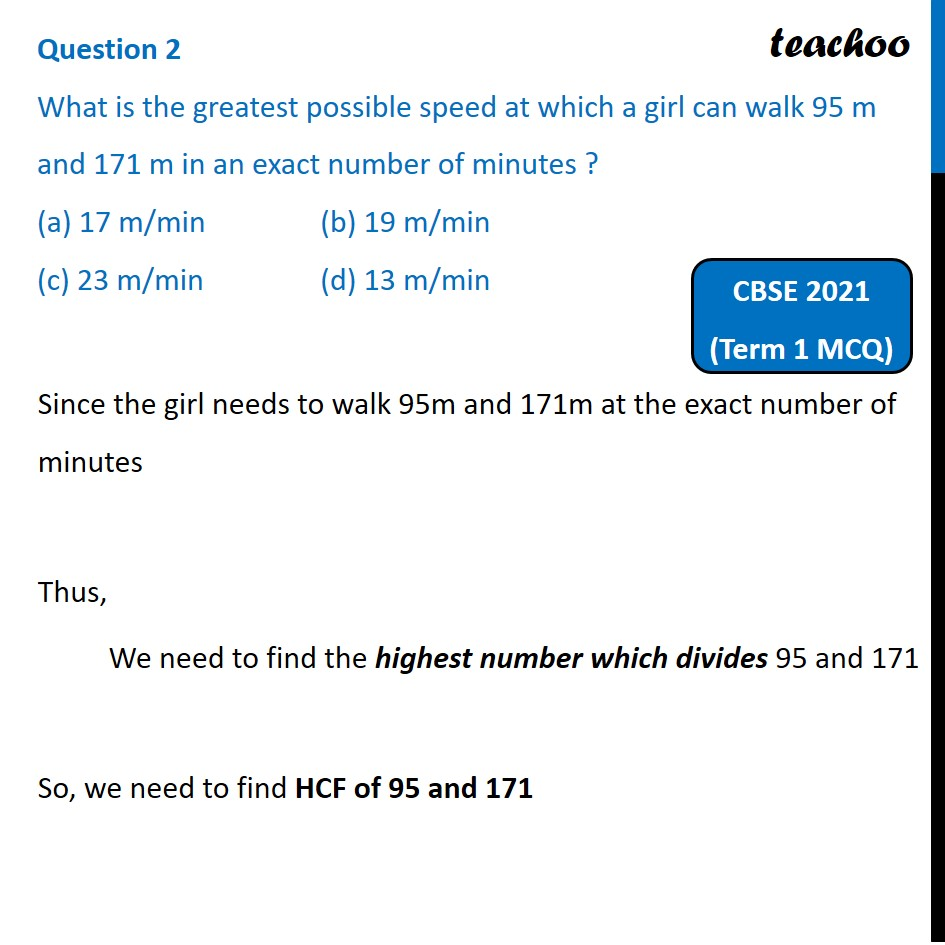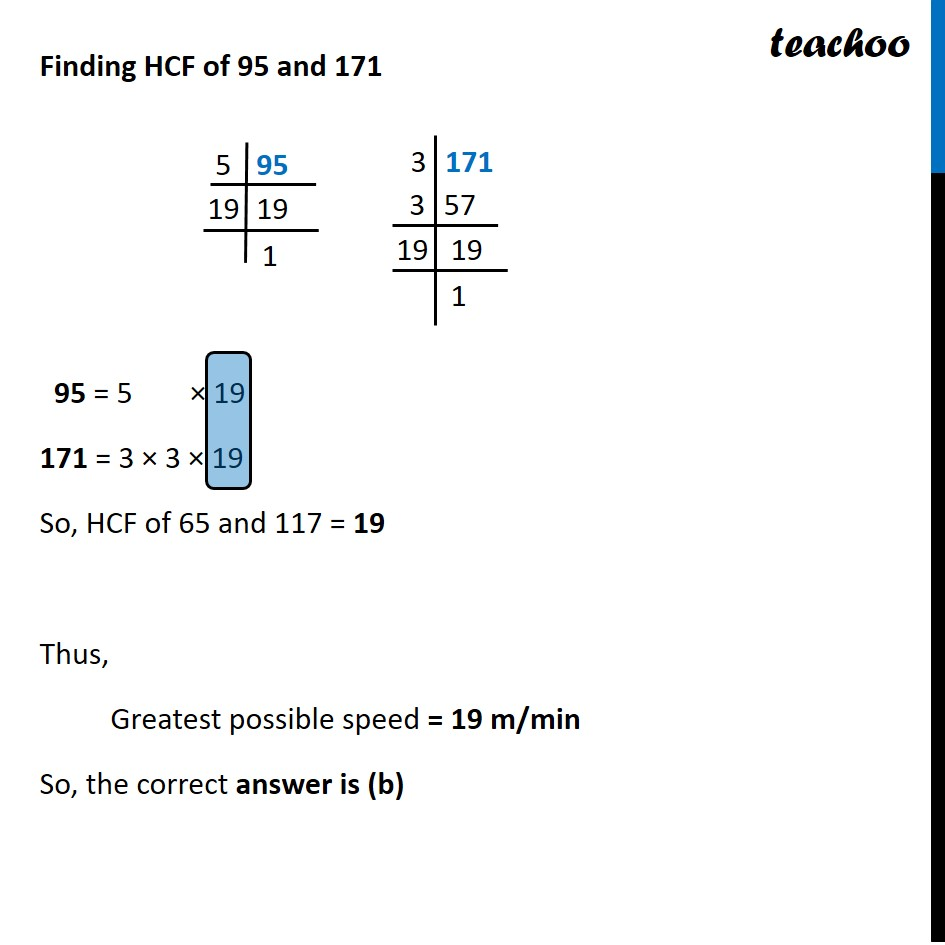Past Year MCQ (Maths Standard)

Chapter 1 Class 10 Real Numbers
Serial order wiseLearn in your speed, with individual attention - Teachoo Maths 1-on-1 Class

### Transcript

Question 2 What is the greatest possible speed at which a girl can walk 95 m and 171 m in an exact number of minutes ? (a) 17 m/min (b) 19 m/min (c) 23 m/min (d) 13 m/minSince the girl needs to walk 95m and 171m at the exact number of minutes Thus, We need to find the highest number which divides 95 and 171 So, we need to find HCF of 95 and 171 Finding HCF of 95 and 171 95 = 5 × 19 171 = 3 × 3 × 19 So, HCF of 65 and 117 = 19 Thus, Greatest possible speed = 19 m/min So, the correct answer is (b) 5 95 19 19 3 171 3 57 19 19 1